# Multiplicatively monotone Euclidean norm admits unique Euclidean division for exact divisor

## Statement

Suppose$R$ is an commutative unital ring with a Euclidean norm$N$ that is multiplicatively monotone: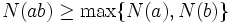$N(ab) \ge \max \{ N(a), N(b) \}$ whenever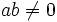$ab \ne 0$.

Suppose$a = bc$ for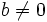$b \ne 0$. Then, we cannot write: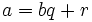$a = bq + r$

with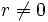$r \ne 0$ and$N(r) < N(b)$.

## Proof

Given: An integral domain$R$ with multiplicatively monotone Euclidean norm$N$.$b \ne 0$ and$a = bc$.

To prove: We cannot write$a = bq + r$ with$N(r) < N(b)$.

Proof: Suppose$a = bq + r$ with$N(r) < N(b)$. Then, since$a = bc$, we get:$bc = bq + r \implies b(c - q) = r$.

Take$N$ both sides, to get:$N(b(c-q)) = N(r)$.

Since$N$ is multiplicatively monotone, we get:$N(b(c-q)) \ge \max \{ N(b), N(c - q) \}$.

On the other hand, we have, by assumption:$N(r) < N(b)$.

This is a contradiction, completing the proof.